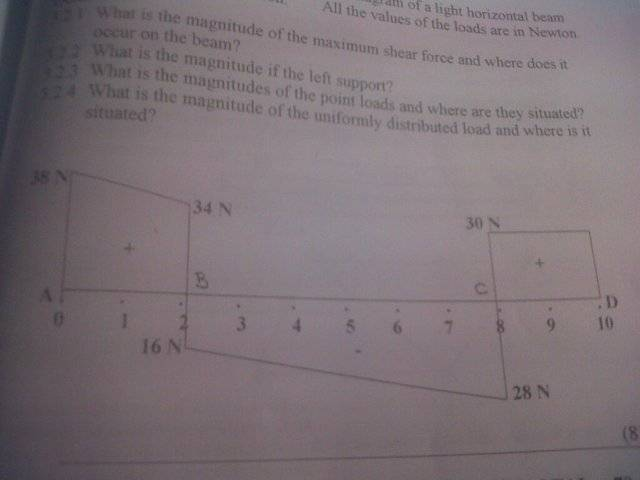# Finding point loads in shear force diagram

Sl1ver

## Homework Statement

I have a shear force diagram and im asked to find the magnitudes of the point loads and where are they situated

My question is, what am I looking for to find the point loads in a shear force diagram?

Mentor

## Homework Statement

I have a shear force diagram and im asked to find the magnitudes of the point loads and where are they situated

My question is, what am I looking for to find the point loads in a shear force diagram?

## The Attempt at a Solution

I assume you are looking at point loading along the length of a beam. How does the shear force change from one side of the point load to the other? Do you have any examples of shear force diagrams in your textbook involving point loads?

Sl1ver
my textbook doesn't realy look at it. i've googled but haven't found much.

here is an example

10 m light horizontal beam
it has points ABCD

A at 0m, B at 2m, c at 8m and D at 10m

it goes positive 38N at A then to 34N at B then straight down to negative 16N the line then goes to C to negative 28N and then straight up to positive 30N and then to D and straight down to 0.

Im just not too sure what exactly to look for to determine where a point is.

Mentor
Do an equilibrium force balance on a tiny section of the beam cut out on either side of one of the point loads. On one side of the cut the shear force is pointing upward, and on the other side of the cut, the shear force is pointing downward. How is the shear force on one side of the cut related to the shear force on the other side of the cut and to the point load?

Sl1ver
here is the diagramMentor
Let me guess. The point loads are at points A, B, C, and D. From what you see on the graph, what is the key characteristic of the shear force variation at these locations?

A. It varies continuously
or
B. It undergoes a jump change discontinuity

Sl1ver
I would go with B on your question.

Mentor
I would go with B on your question.

Right. Now how are these jump changes in shear force related to the point loads? Hint: see my first reply.

Sl1ver
To be honest im not too sure.

Mentor
Imagine that you cut out a small section of the beam on either side of one of the point loads, and replace the internal loading that was present on the two faces of the section with a tensile force, a moment, and a shear force. This tensile force, moment, and shear force are equal to the corresponding quantities within the loaded beam before the cut was made. Now treat the cut-out section as a free body, and perform an equilibrium force balance on the section in the vertical direction. From this equilibrium force balance, you should be able to determine the jump change in the shear force from one side of the section to the other.